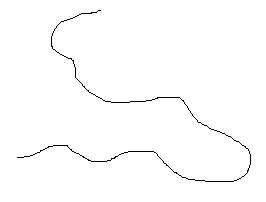# Polymer Chemistry: Topology

$$\newcommand{\vecs}{\overset { \rightharpoonup} {\mathbf{#1}} }$$ $$\newcommand{\vecd}{\overset{-\!-\!\rightharpoonup}{\vphantom{a}\smash {#1}}}$$$$\newcommand{\id}{\mathrm{id}}$$ $$\newcommand{\Span}{\mathrm{span}}$$ $$\newcommand{\kernel}{\mathrm{null}\,}$$ $$\newcommand{\range}{\mathrm{range}\,}$$ $$\newcommand{\RealPart}{\mathrm{Re}}$$ $$\newcommand{\ImaginaryPart}{\mathrm{Im}}$$ $$\newcommand{\Argument}{\mathrm{Arg}}$$ $$\newcommand{\norm}{\| #1 \|}$$ $$\newcommand{\inner}{\langle #1, #2 \rangle}$$ $$\newcommand{\Span}{\mathrm{span}}$$ $$\newcommand{\id}{\mathrm{id}}$$ $$\newcommand{\Span}{\mathrm{span}}$$ $$\newcommand{\kernel}{\mathrm{null}\,}$$ $$\newcommand{\range}{\mathrm{range}\,}$$ $$\newcommand{\RealPart}{\mathrm{Re}}$$ $$\newcommand{\ImaginaryPart}{\mathrm{Im}}$$ $$\newcommand{\Argument}{\mathrm{Arg}}$$ $$\newcommand{\norm}{\| #1 \|}$$ $$\newcommand{\inner}{\langle #1, #2 \rangle}$$ $$\newcommand{\Span}{\mathrm{span}}$$$$\newcommand{\AA}{\unicode[.8,0]{x212B}}$$

Polymers are very large molecules with unusual physical properties that depend on the interactions between its chains. An important factor in these interactions is the shape of the chain making up the backbone of the molecule.

## Linear Polymers

Some polymer molecules are linear, similar to a normal alkane such as n-decane. An example is high density polyethylene (HDPE), which can contain more than 1000 CH2 groups.Simulated structures of HDPE are shown below. The skeletal structure shows only the polymer backbone.

Polymer Simulated Structure Simulated Skeletal Structure
LinearHDPE has a high density because the linear molecules can pack closely.

## Nonlinear (Branched) Polymers

Some polymers, such as low density polyethylene (LDPE), have branches of different sizes irregularly spaced along the chain. Such polymers are said to be nonlinear. Polymers with pendant groups, such as the methyl group in polypropylene, are considered to be linear.

Polymer Simulated Structure Simulated Skeletal Structure
NonlinearThe branches prevent the nonlinear molecules from packing as closely as the linear, reducing their density.

## Network Polymers

Some polymers have cross-links between polymer chains creating three-dimensional networks. A high density of cross-linking restricts the motion of the chains and leads to a rigid material. A simulated skeletal structure of a network polymer with a high cross-link density is shown below.A simulated skeletal structure of a network polymer